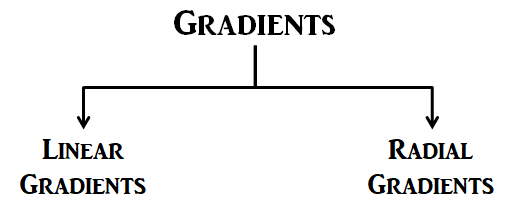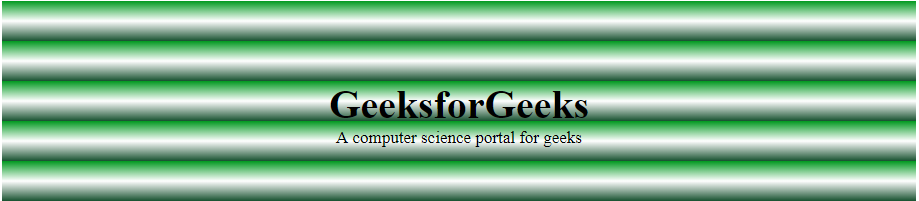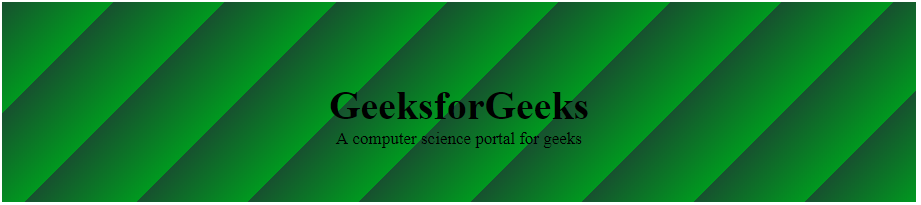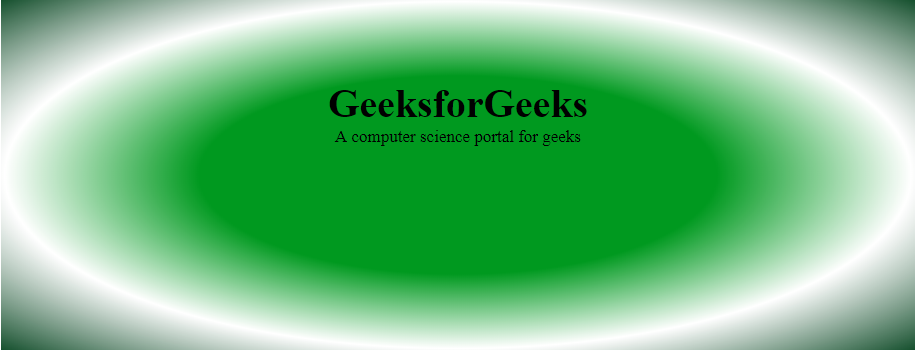Related Articles
CSS | Gradients
• Last Updated : 04 Dec, 2018

CSS is the mechanism to adding style in the various web documents. One of the important features of CSS includes Gradients. Gradients allows to display smooth transitions between two or more colors.There are two types of Gradients.Linear Gradients: It includes the smooth color transitions to going up, down, left, right and diagonally. The minimum two color required to create a linear gradient. More then two color elements can be possible in linear gradients. The starting point and the direction is needed for the gradient effect.

Syntax:

`background-image: linear-gradient(direction, color-stop1, color-stop2, ...);`
• Top to Bottom: In this image, the transition started to white color and ended with green color. On exchanging the color sequence, the transition will start with green and will end with white.
Example:
 ```<``html``>``    ``<``head``>``        ``<``title``>CSS Gradients``        ``<``style``>``            ``#main {``                ``height: 200px;``                ``background-color: white;``                ``background-image: linear-gradient(white, green); ``            ``}``            ``.gfg {``                ``text-align:center;``                ``font-size:40px;``                ``font-weight:bold;``                ``padding-top:80px;``            ``}``            ``.geeks {``                ``font-size:17px;``                ``text-align:center;``            ``}``        ````    ````    ``<``body``>``        ``<``div` `id``=``"main"``>``            ``<``div` `class` `= ``"gfg"``>GeeksforGeeks``            ``<``div` `class` `= ``"geeks"``>A computer science portal for geeks``        ````    ````                    `

Output:• Left to Right: In this image, the transition started from left to right. It starts from white transitioning to green.
Example:

 ```<``html``>``    ``<``head``>``        ``<``title``>CSS Gradients``        ``<``style``>``            ``#main {``                ``height: 200px;``                ``background-color: white;``                ``background-image: linear-gradient(to right, white, green); ``                ``}``            ``.gfg {``                ``text-align:center;``                ``font-size:40px;``                ``font-weight:bold;``                ``padding-top:80px;``            ``}``            ``.geeks {``                ``font-size:17px;``                ``text-align:center;``            ``}``        ````    ````    ``<``body``>``        ``<``div` `id``=``"main"``>``            ``<``div` `class` `= ``"gfg"``>GeeksforGeeks``            ``<``div` `class` `= ``"geeks"``>A computer science portal for geeks``        ````    ````                    `

Output:• Diagonal: This transition started from top-left to bottom-right. It starts with green transition to white. For the diagonal gradient, need to specify both horizontal and vertical starting positions.
Example:
 ```<``html``>``    ``<``head``>``        ``<``title``>CSS Gradients``        ``<``style``>``            ``#main {``                ``height: 200px;``                ``background-color: white; ``                ``background-image: linear-gradient(to bottom right,``                                ``green, rgba(183, 223, 182, 0.4)); ``            ``}``            ``.gfg {``                ``text-align:center;``                ``font-size:40px;``                ``font-weight:bold;``                ``padding-top:80px;``            ``}``            ``.geeks {``                ``font-size:17px;``                ``text-align:center;``            ``}``        ````    ````    ``<``body``>``        ``<``div` `id``=``"main"``>``            ``<``div` `class` `= ``"gfg"``>GeeksforGeeks``            ``<``div` `class` `= ``"geeks"``>A computer science portal for geeks``        ````    ````                    `

Output:• Repeating Linear Gradient: CSS allows the user to implement multiple linear gradients using a single function repeating-linear-gradient(). The image here contains 3 colors in each transition with some percentage value.
Example:
 ```<``html``>``    ``<``head``>``        ``<``title``>CSS Gradients``        ``<``style``>``            ``#main {``                ``height: 200px;``                ``background-color: white; ``                ``background-image: repeating-linear-gradient(#090,``                                         ``#fff 10%, #2a4f32 20%);``            ``}``            ``.gfg {``                ``text-align:center;``                ``font-size:40px;``                ``font-weight:bold;``                ``padding-top:80px;``            ``}``            ``.geeks {``                ``font-size:17px;``                ``text-align:center;``            ``}``        ````    ````    ``<``body``>``        ``<``div` `id``=``"main"``>``            ``<``div` `class` `= ``"gfg"``>GeeksforGeeks``            ``<``div` `class` `= ``"geeks"``>A computer science portal for geeks``        ````    ````                    `

Output:• Angles on Linear Gradients: CSS allows the user to implement directions in Linear Gradients rather than restricting themselves to predefined directions.
Example:
 ```<``html``>``    ``<``head``>``        ``<``title``>CSS Gradients``        ``<``style``>``            ``#main {``                ``height: 200px;``                ``background-color: white; ``                ``background-image: repeating-linear-gradient(-45deg,``                                                ``#090, #2a4f32 10%);``            ``}``            ``.gfg {``                ``text-align:center;``                ``font-size:40px;``                ``font-weight:bold;``                ``padding-top:80px;``            ``}``            ``.geeks {``                ``font-size:17px;``                ``text-align:center;``            ``}``        ````    ````    ``<``body``>``        ``<``div` `id``=``"main"``>``            ``<``div` `class` `= ``"gfg"``>GeeksforGeeks``            ``<``div` `class` `= ``"geeks"``>A computer science portal for geeks``        ````    ````                    `

Output:CSS Radial Gradients: A radial gradient differs from a linear gradient. It starts at a single point and emanates outward. By default, the first color starts at the center position of the element and then fade to the end color towards the edge of the element. Fade happens at an equal rate until specified.
Syntax:

`background-image: radial-gradient(shape size at position, start-color, ..., last-color);`
• Radial Gradient- evenly spaced color stops: In CSS, by default, the fade happens at an equal rate. The following figure shows the Radial Gradient with even color stops.
Example:
 ```<``html``>``    ``<``head``>``        ``<``title``>CSS Gradients``        ``<``style``>``            ``#main {``                ``height: 350px;``                ``width: 700px;``                ``background-color: white;``                ``background-image: radial-gradient(#090, #fff, #2a4f32);``            ``}``            ``.gfg {``                ``text-align:center;``                ``font-size:40px;``                ``font-weight:bold;``                ``padding-top:80px;``            ``}``            ``.geeks {``                ``font-size:17px;``                ``text-align:center;``            ``}``        ````    ````    ``<``body``>``        ``<``div` `id``=``"main"``>``            ``<``div` `class` `= ``"gfg"``>GeeksforGeeks``            ``<``div` `class` `= ``"geeks"``>A computer science portal for geeks``        ````    ````                    `

Output:• Radial Gradient- unevenly spaced color stops: CSS allows the user to have variation in spacing of color stops while applying radial gradient feature.
Example:
 ```<``html``>``    ``<``head``>``        ``<``title``>CSS Gradients``        ``<``style``>``            ``#main {``                ``height: 350px;``                ``width: 100%;``                ``background-color: white;``                ``background-image: radial-gradient(#090 40%, #fff, #2a4f32);``            ``}``            ``.gfg {``                ``text-align:center;``                ``font-size:40px;``                ``font-weight:bold;``                ``padding-top:80px;``            ``}``            ``.geeks {``                ``font-size:17px;``                ``text-align:center;``            ``}``        ````    ````    ``<``body``>``        ``<``div` `id``=``"main"``>``            ``<``div` `class` `= ``"gfg"``>GeeksforGeeks``            ``<``div` `class` `= ``"geeks"``>A computer science portal for geeks``        ````    ````                    `

Output:My Personal Notes arrow_drop_up